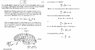# Flux rule for motional emf

• aaaa202
In summary, the flux rule for motional emf is d\phi/dt = -\epsilon, where d\phi/dt is the change in magnetic flux over time and \epsilon is the induced emf. One may find this rule intuitive by understanding Lenz's law, which is a qualitative version of the flux rule. The proof of this rule provided in the attached book uses the definition of flux and the Lorentz force law, which may appear earlier in the text.

#### aaaa202

The flux rule for motional emf can be stated as:

d$\phi$/dt = -$\epsilon$

I have some questions regarding this. 1) Should I find this rule intuitive?
And secondly a proof of this rule is given in my book, which I have attached. I don't really understand the idea of the proof - is the idea to proof the above rule or to just proof that if it works, it will do so for loops, which "do not even maintain a fixed shape". Because the words seem to suggest the later but on the other hand that would mean that the above rule is generally unproved in my book.

#### Attachments

•fluxrule.jpg
30.6 KB · Views: 741
aaaa202 said:
The flux rule for motional emf can be stated as:

d$\phi$/dt = -$\epsilon$

I have some questions regarding this. 1) Should I find this rule intuitive?[..]
Just answering 1: You may find it intuitive by means of Lenz's law, which is a qualitative version of the above. It has similarity to Newton's third law, in the sense of action is reaction.

okay but Lenz' law is just a more qualitative statement of the flux rule - but perhaps there isn't more to it.
What about 2)? That one was more of a puzzle to me.

aaaa202 said:
okay but Lenz' law is just a more qualitative statement of the flux rule - but perhaps there isn't more to it.
What about 2)? That one was more of a puzzle to me.
It seems to prove the flux rule from their definition of flux plus the Lorentz force law. Both should appear earlier in the text.

## 1. What is the Flux rule for motional emf?

The Flux rule for motional emf, also known as Faraday's Law, states that a changing magnetic flux through a loop of wire will induce an emf (electromotive force) in the loop. This emf will create a current in the wire if there is a complete circuit.

## 2. How is the Flux rule for motional emf calculated?

The Flux rule for motional emf is calculated by taking the derivative of the magnetic flux with respect to time. In mathematical terms, it can be expressed as: emf = -N * dΦ/dt, where N is the number of turns in the loop and Φ is the magnetic flux.

## 3. What is the significance of the negative sign in the Flux rule for motional emf?

The negative sign in the Flux rule for motional emf indicates the direction of the induced current. It follows Lenz's Law, which states that the induced current will flow in a direction that opposes the change in magnetic flux.

## 4. Can the Flux rule for motional emf be applied to any type of circuit?

Yes, the Flux rule for motional emf can be applied to any type of circuit as long as there is a changing magnetic flux through the loop. This includes both AC and DC circuits.

## 5. What are some real-life applications of the Flux rule for motional emf?

The Flux rule for motional emf has many practical applications, including generators, transformers, and induction cooktops. It also plays a crucial role in the functioning of electric motors, which convert electrical energy into mechanical energy based on the Flux rule for motional emf.